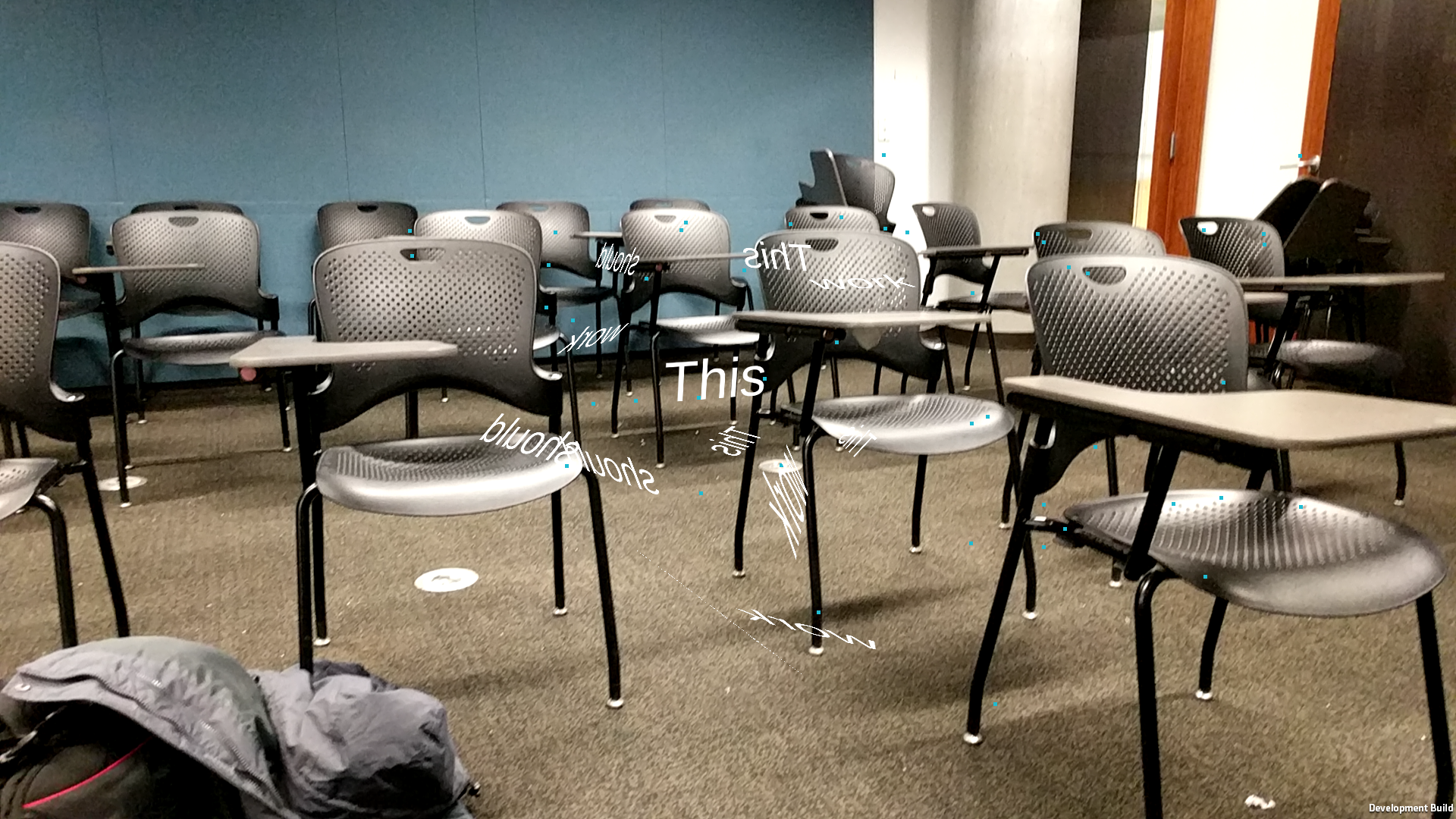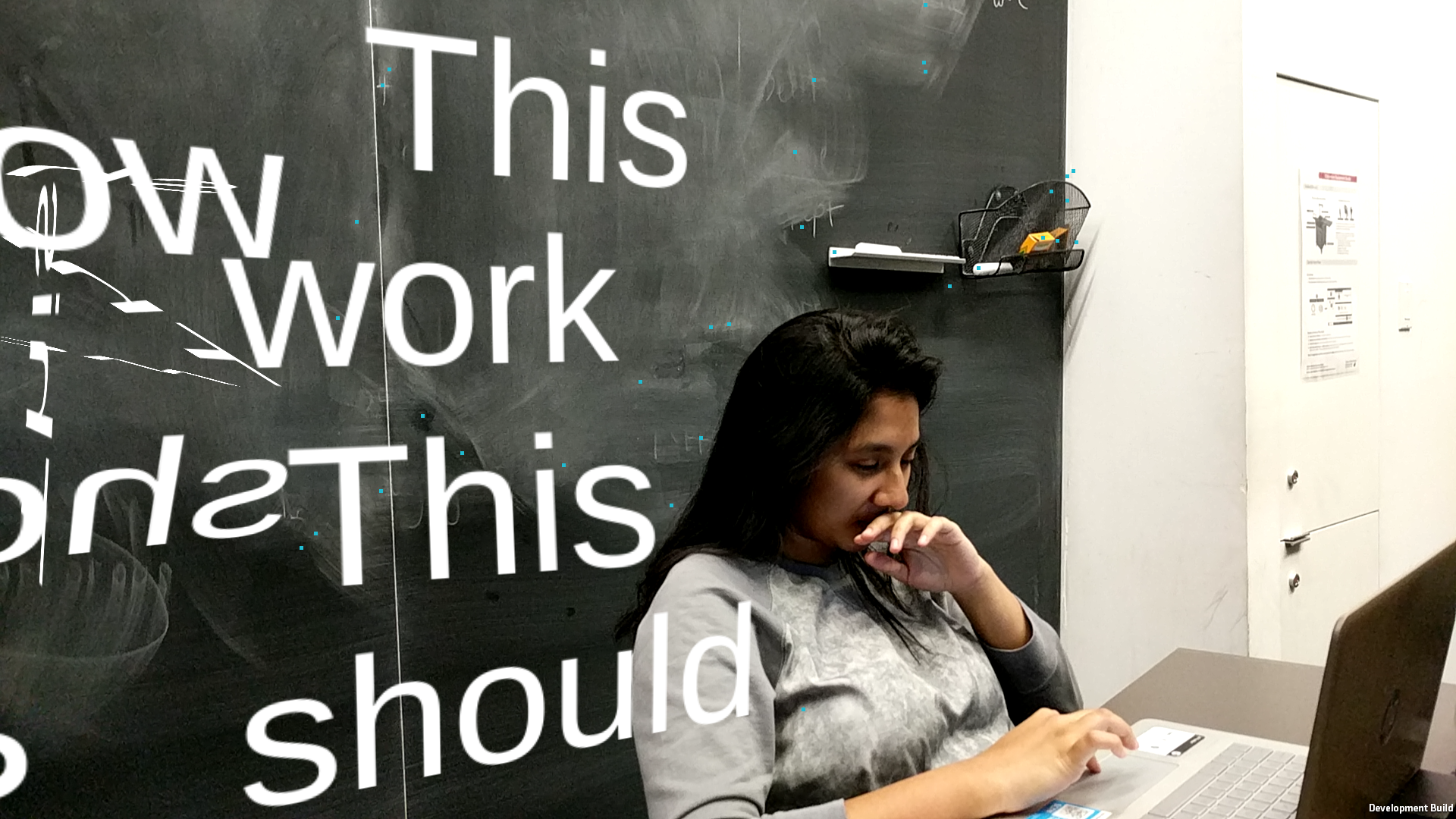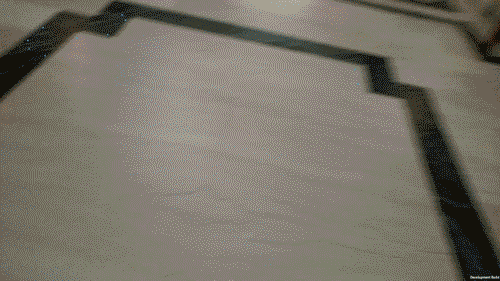# farcar – ArProject

The biggest technical problem in my project was getting wall detection to work for Google AR Core: something that I wasn’t able to solve last week. I had spent so much time trying to figure our how to generate TrackingPlanes when in reality I could have just used the Point Cloud data I was getting and track objects to it. And that’s exactly what I did.The algorithm works like this:

Computer Language

• Save an array ‘Content’ of String values.
• Read from the point cloud data (Points are in the form <x,y,z,c> where c is the confidence).
• If screen is touched, read from the touch data.
• Increment ‘touchCount’.
• Assign Content[touchCount % Content.Count] to a 3D text layer (this will be used later when we actually place down objects).
• Project all the 3D points onto a 2D screen.
• Using the <x,y> position from the touch data, find the closest 2D projected 3D point.
• Find the two closest points to that point in 3D space.
• Construct two vectors out of the three points (this is our plane).
• Get the cross product of the two vectors.
• Using the initial 3D point with the cross product, instantiate a new 3D text layer with the position of the 3D point and rotation of the cross product.

Human Language

• Press somewhere on the screen
• The closest point to where you touched will be the location a new text object will be generated.
• The text will try to orient to the surface by extrapolating a plane based on its neighboring points

After some additional refining (such as point filtering for low confidence values) I was able to get more accurate results.Code

```using System.Collections; using System.Collections.Generic; using UnityEngine; using UnityEngine.UI; using GoogleARCore.HelloAR;   public class TextCore : MonoBehaviour {   public int clickCount; //number of screen taps public TextMesh textLayer; //text layer to be changed public GameObject TrackedPointPrefab; //prefab that generates over tracked points onscreen public GameObject GeneratePointPrefab; //prefab that generates over a particular tracked point when screen is tapped public List points; //array of tracked points &lt;x,y,z,c&gt; s.t. c is confidence from 0 to 1   public Camera Camera;   List content = new List(); //array of words to process   void filterPoints(float filter) { for(var i = 0; i &lt; points.Count; i++) { if(points[i].w &lt; filter) points.RemoveAt(i); } } //canSee (camera, point) = true if point is in view of camera, false otherwise bool canSee (Camera camera, Vector3 point) { Vector3 viewportPoint = camera.WorldToViewportPoint(point); return (viewportPoint.z &gt; 0 &amp;&amp; (new Rect(0, 0, 1, 1)).Contains(viewportPoint)); }   //minIndxFinder (minIndx, minReg, touchPos) = the index i s.t points[i] is the closest point to touchPos int minIndxFinder(int minIndx, float minReg, Vector3 touchPos) { for(int i = 0; i &lt; points.Count; i++) { if(canSee(Camera, new Vector3( points[i].x, points[i].y, points[i].z))) { Vector3 pointTemp = Camera.WorldToViewportPoint(new Vector3( points[i].x, points[i].y, points[i].z)); Vector3 pointTempAdj = new Vector3( (Screen.width) * pointTemp.x, (Screen.height) * pointTemp.y, 0);   float minRegTemp = (pointTempAdj - touchPos).magnitude; if(minRegTemp &lt; minReg) { minReg = minRegTemp; minIndx = i; } } } return minIndx; }   void generateText (Vector3 target, Vector3 cross, int depthLen) {   Vector3 camDir = Camera.transform.forward; float dot = Vector3.Dot(camDir, cross); if(dot &lt; 0) { cross = Vector3.Reflect(camDir, cross); }   float dist = ((Camera.transform.position) - cross).magnitude; textLayer.fontSize = 1000 + 1000 * (int)dist;   textLayer.color = Color.black; Vector3 depth = target; for(int i = 0; i &lt; depthLen; i++) { if(i &gt; depthLen - 5) { textLayer.color = Color.white; } Instantiate(GeneratePointPrefab, depth, Quaternion.LookRotation(cross, new Vector3 (0,1,0)), transform); depth -= 0.001f*cross; } }   void updateTouch() { if (Input.touchCount &gt; 0) { Touch touch = Input.GetTouch(0); if(touch.phase == TouchPhase.Began) { float x = touch.position.x; float y = touch.position.y; Vector3 touchPos = new Vector3(x, y, 0.0f); clickCount++; clickCount = clickCount % content.Count; textLayer.text = content[clickCount];   if(points.Count &gt; 2) { int minIndx = minIndxFinder(0, 99999999999999f, touchPos); int minIndx2 = 1; int minIndx3 = 2; float minDis1 = 99999999999999f; float minDis2 = 99999999999999f; for(int i = 0; i &lt; points.Count; i++) { if(i != minIndx) { float minDisTemp = (points[minIndx] - points[i]).magnitude; if(minDisTemp &lt; minDis1) { minDis1 = minDisTemp; minIndx2 = i; } else if(minDisTemp &lt; minDis2) { minDis2 = minDisTemp; minIndx3 = i; } } } Vector3 v1 = points[minIndx2] - points[minIndx]; Vector3 v2 = points[minIndx3] - points[minIndx]; Vector3 cross = Vector3.Cross(v1, v2); cross /= cross.magnitude; Vector3 target = new Vector3(points[minIndx].x, points[minIndx].y, points[minIndx].z); generateText(target, cross, 25); } } } }   void Start() { content.Add("Now it's time \n to go to bed"); }   void Update() { points = GetComponent().getPoints();   print(Camera.transform.forward);   //filterPoints(0.1);   updateTouch(); } }```

Thanks to Golan & friends of the Studio for helping me mathematically plan the project.